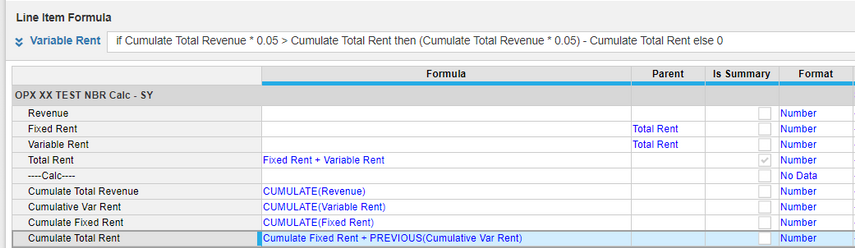# Circular Reference with CUMULATEHi All,

I am trying to solve an excel model in Anaplan where my current value is a calculation of the cumulative of previous values. The calculation works fine in excel but I am unable to break this down in Anaplan. I am adding the screenshot for the logic of this model below. Any help or suggestions would be helpful.Tagged:

•these formulas don't work ?

PREVIOUS() is how we avoid circular references in Anaplan world but I don't see any in what you are doing here
•Hi,

I have found that circular references can emerge when you daisy chain the calculations and if you run the calculations in parallel rather than in series you should be able to resolve them.

In your case take each element of the final calculation and use the formulas rather than the line items. Therefore, the calculation is independent and the circular reference should be broken.

Good luck,

Chris

•Hi Nathan,

I tried using PREVIOUS() while calculating total rent. However, with CUMULATE() variable rent, it is not working as expected. I have also tried other combinations of formulas using PREVIOUS() and CUMULATE() but it did not work for me.
•Hi Chris,

I tried to do as per your suggestions as shown below. My understanding is that with CUMULATE() & PREVIOUS() variable rent, it is referring back to the same line item and is unable to break circular reference.

if cumulate(Revenue) *0.05> cumulate(Previous Variable Rent)+cumulate(Fixed Rent) then cumulate(Revenue) *0.05- (cumulate(Previous Variable Rent)+cumulate(Fixed Rent)) else 0

•I reviewed the formula and came up with the following;

IF CUMULATE(Revenue) * 0.05 > (CUMULATE(Fixed Rent) + PREVIOUS(CUMULATE(Var Rent))) THEN (CUMULATE(Total Revenue) * 0.05 ) - (CUMULATE(Fixed Rent) + PREVIOUS(CUMULATE(Var Rent))) ELSE 0

I think the issue is with the final line item; Cumulate Total Rent.

Try breaking the series with this one and restate it as; (CUMULATE(Fixed Rent) + PREVIOUS(CUMULATE(Var Rent)))

•For optimal performance, try breaking that calculation up so it is not doing all of the cumulates for the line items for every cell in this line item.  Also, when any of those line items change (Revenue, Fixed Rent, Var Rent, or Total Revenue) change, this entire logic gets kicked off (for ever cell of this line item) which is why breaking it up it much better from a performance perspective.  Additionally, in the above, you are doing a cumulate of Fix Rent multiple times.  Again, if you break this up, you are only doing it once.

Rob